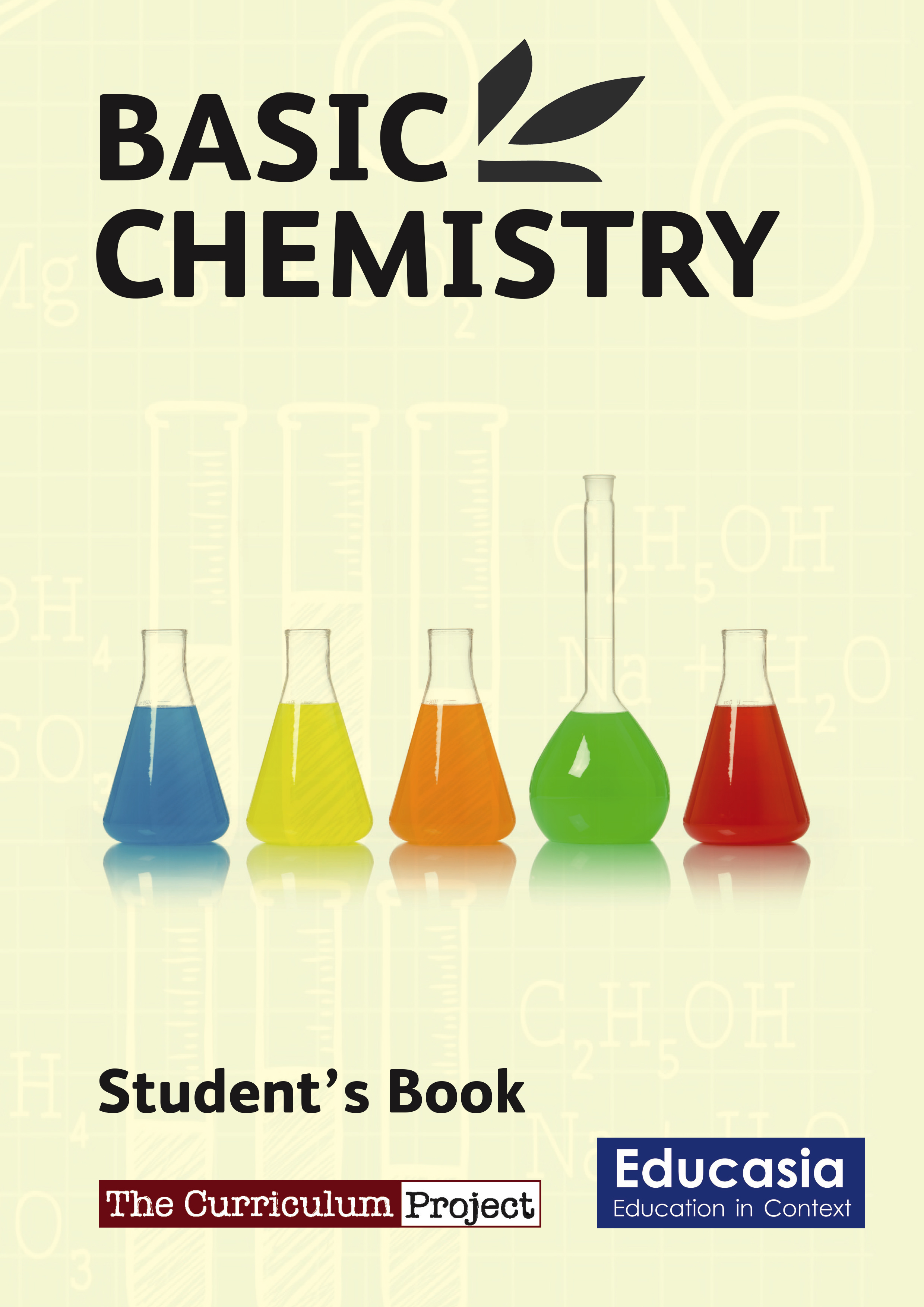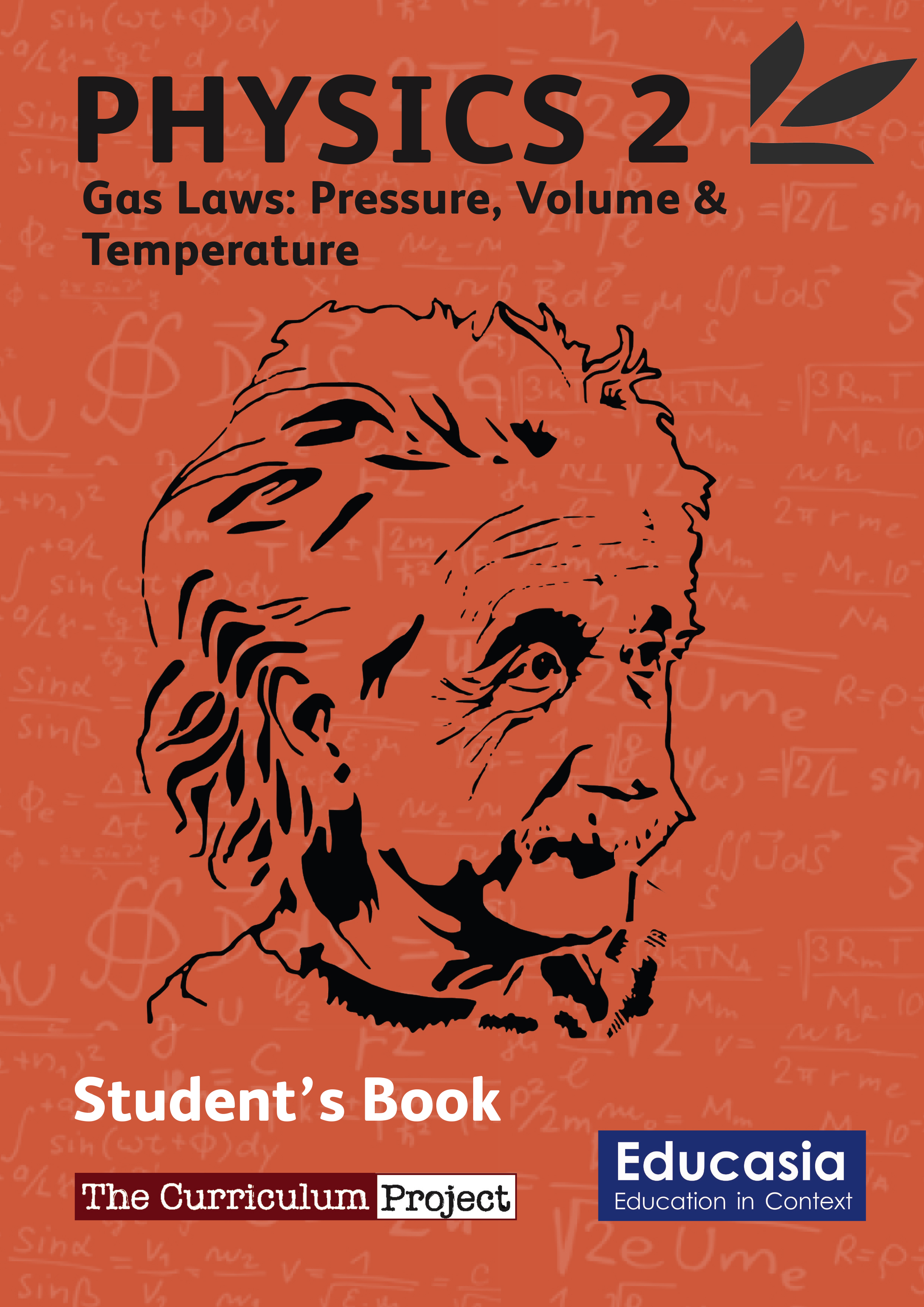## Maths and Science

###### Maths Modules

These modules make a one-year post-secondary maths course. They consist of an English language Student’s Book and Teacher’s Guide.

######Maths Module 1: Numbers

Some of the topics covered in the module are a review of basic arithmetic, fractions, ratio, percentages and indices.

######Maths Module 2: Algebra

It is designed to review basic algebra before moving to quadratic equations, sequences and the laws of indices.

######Maths Module 3: Statistics

It covers all the topics needed to teach students how to present and interpret data. Students will create different charts and graphs and use standard techniques to draw conclusions and make predictions.

######Maths Module 4: Geometry

It covers Geometry and Trigonometry, which are extremely useful in design, science, architecture, and engineering.

###### Science Series

These modules consist of and English language Student’s Book and Teacher’s Book.

###### Basic ChemistryThis introduces basic chemistry concepts such as matter and particles, acids/bases and chemical reactions.

######Physics 1: Atoms & Molecules

This module looks at the main laws and rules affecting atoms and molecules.

######Physics 2: Gas Laws: Pressure, Volume & Temperature

This module reviews gas laws with respect to pressure, volume, temperature and amount.

######Physics 3: Motion & Force

This module explains the main concepts related to motion and force, including gravity, pressure, speed and friction.